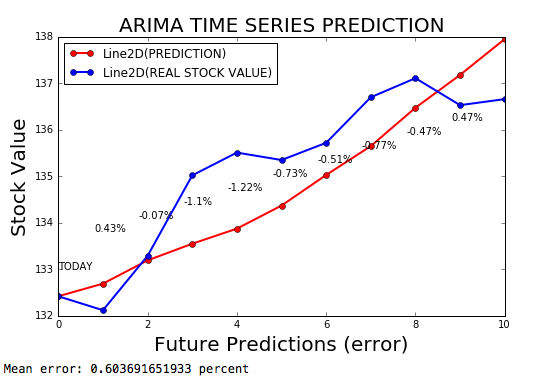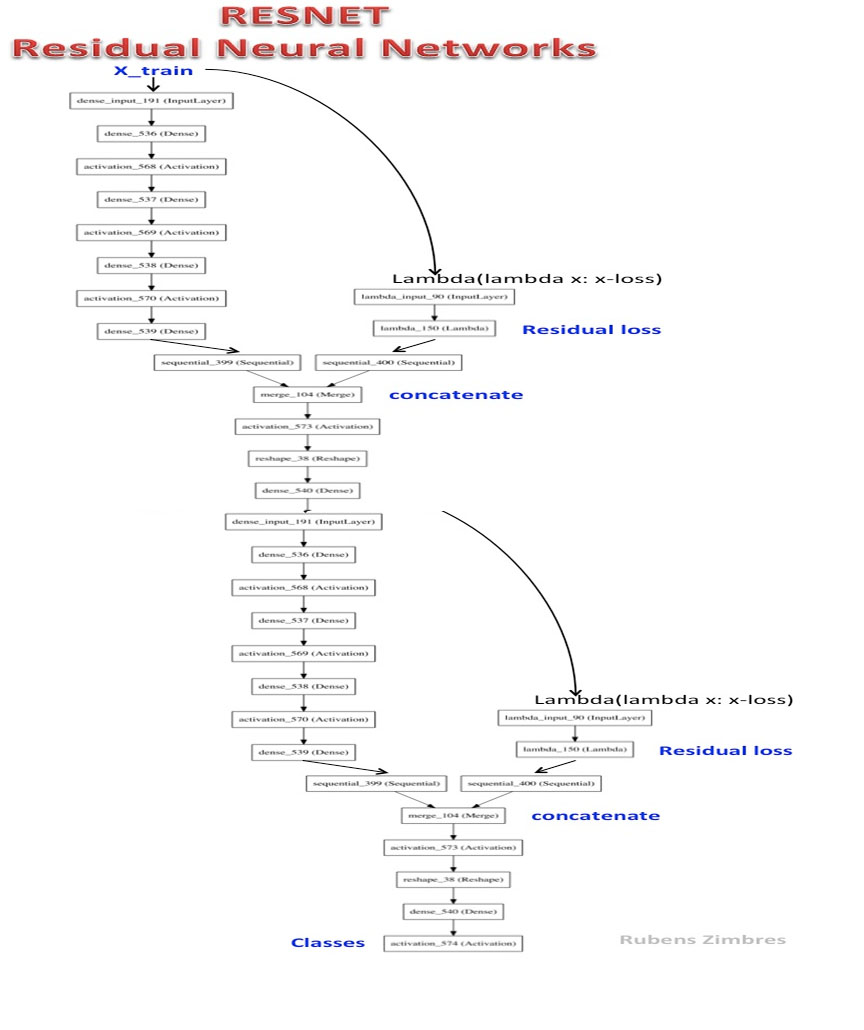# Repo 2017

Python codes in Machine Learning, NLP, Deep Learning and Reinforcement Learning with Keras and Theano
Alternatives To Repo 2017
Data Science Ipython Notebooks25,025
a month ago33otherPython
Data science Python notebooks: Deep learning (TensorFlow, Theano, Caffe, Keras), scikit-learn, Kaggle, big data (Spark, Hadoop MapReduce, HDFS), matplotlib, pandas, NumPy, SciPy, Python essentials, AWS, and various command lines.
Deepo6,312
4 months ago1mitPython
Setup and customize deep learning environment in seconds.
Keras Rl5,3485126 months ago7June 01, 201843mitPython
Deep Reinforcement Learning for Keras.
Deep Learning Keras Tensorflow2,863
2 years ago12mitJupyter Notebook
Introduction to Deep Neural Networks with Keras and Tensorflow
Keras Vis2,5922813 years ago11July 06, 2017104mitPython
Neural network visualization toolkit for keras
Deepjazz2,583
4 years ago12apache-2.0Python
Deep learning driven jazz generation using Keras & Theano!
Keras Contrib1,582
8 months ago176mitPython
Keras community contributions
Merlin1,189
3 years ago185otherPython
This is now the official location of the Merlin project.
Repo 20171,146
2 years ago1Python
Python codes in Machine Learning, NLP, Deep Learning and Reinforcement Learning with Keras and Theano
Cnngesturerecognizer895
4 months ago12mitPython
Gesture recognition via CNN. Implemented in Keras + Tensorflow/Theano + OpenCV
Alternatives To Repo 2017
Select To Compare

Alternative Project Comparisons

# Python Codes in Data Science

Codes in NLP, Deep Learning, Reinforcement Learning and Artificial Intelligence

Welcome to my GitHub repo.

I am a Data Scientist and I code in R, Python and Wolfram Mathematica. Here you will find some Machine Learning, Deep Learning, Natural Language Processing and Artificial Intelligence models I developed.

Keras version used in models: keras==1.1.0

Autoencoder for Audio is a model where I compressed an audio file and used Autoencoder to reconstruct the audio file, for use in phoneme classification.

Collaborative Filtering is a Recommender System where the algorithm predicts a movie review based on genre of movie and similarity among people who watched the same movie.

Convolutional NN Lasagne is a Convolutional Neural Network model in Lasagne to solve the MNIST task.

Ensembled Machine Learning is a .py file where 7 Machine Learning algorithms are used in a classification task with 3 classes and all possible hyperparameters of each algorithm are adjusted. Iris dataset of scikit-learn.Hyperparameter Tuning RL is a model where hyperparameters of Neural Networks are adjusted via Reinforcement Learning. According to a reward, hyperparameter tuning (environment) is changed through a policy (mechanization of knowledge) using the Boston Dataset. Hyperparameters tuned are: learning rate, epochs, decay, momentum, number of hidden layers and nodes and initial weights.

Keras Regularization L2 is a Neural Network model for regression made with Keras where a L2 regularization was applied to prevent overfitting.

Lasagne Neural Nets Regression is a Neural Network model based in Theano and Lasagne, that makes a linear regression with a continuous target variable and reaches 99.4% accuracy. It uses the DadosTeseLogit.csv sample file.

Lasagne Neural Nets + Weights is a Neural Network model based in Theano and Lasagne, where is possible to visualize weights between X1 and X2 to hidden layer. Can also be adapted to visualize weights between hidden layer and output. It uses the DadosTeseLogit.csv sample file.

Multinomial Regression is a regression model where target variable has 3 classes.

Neural Networks for Regression shows multiple solutions for a regression problem, solved with sklearn, Keras, Theano and Lasagne. It uses the Boston dataset sample file from sklearn and reaches more than 98% accuracy.NLP + Naive Bayes Classifier is a model where movie reviews were labeled as positive and negative and the algorithm then classifies a totally new set of reviews using Logistic Regression, Decision Trees and Naive Bayes, reaching an accuracy of 92%.

NLP Anger Analysis is a Doc2Vec model associated with Word2Vec model to analyze level of anger using synonyms in consumer complaints of a U.S. retailer in Facebook posts.

NLP Consumer Complaint is a model where Facebook posts of a U.S. computer retailer were scraped, tokenized, lemmatized and applied Word2Vec. After that, t-SNE and Latent Dirichlet Allocation were developed in order to classify the arguments and weights of each keyword used by a consumer in his complaint. The code also analyzes frequency of words in 100 posts.

NLP Convolutional Neural Network is a Convolutional Neural Network for Text in order to classify movie reviews.

NLP Doc2Vec is a Natural Language Procesing file where cosine similarity among phrases is measured through Doc2Vec.

NLP Document Classification is a code for Document Classification according to Latent Dirichlet Allocation.

NLP Facebook Analysis analyzes Facebook posts regarding Word Frequency and Topic Modelling using LDA.

NLP Facebook Scrap is a Python code for scraping data from Facebook.

NLP - Latent Dirichlet Allocation is a Natural Language Processing model where a Wikipedia page on Statistical Inference is classified regarding topics, using Latent Dirichlet Allocation with Gensim, NLTK, t-SNE and K-Means.

NLP Probabilistic ANN is a Natural Language Processing model where sentences are vectorized by Gensim and a probabilistic Neural Network model is deveoped using Gensim, for sentiment analysis.

NLP Semantic Doc2Vec + Neural Network is a model where positive and negative movie reviews were extracted and semantically classified with NLTK and BeautifulSoup, then labeled as positive or negative. Text was then used as an input for the Neural Network model training. After training, new sentences are entered in the Keras Neural Network model and then classified. It uses the zip file.

NLP Sentiment Positive is a model that identifies website content as positive, neutral or negative using BeautifulSoup and NLTK libraries, plotting the results.

NLP Twitter Analysis ID # is a model that extracts posts from Twitter based in ID of user or Hashtag.

NLP Twitter Scrap is a model that scraps Twitter data and shows the cleaned text as output.

NLP Twitter Streaming is a model of analysis of real-time data from Twitter (under development).

NLP Twitter Streaming Mood is a model where the evolution of mood Twitter posts is measured during a period of time.

NLP Wikipedia Summarization is a Python code that summarizes any given page in a few sentences.

NLP Word Frequency is a model that calculates the frequency of nouns, verbs, words in Facebook posts.

Probabilistic Neural Network is a Probabilistic Neural Network for Time Series Prediction.

REAL-TIME Twitter Analysis is a model where Twitter streaming is extracted, words and sentences tokenized, word embeddings were created, topic modeling was made and classified using K-Means. Then, NLTK SentimentAnalyzer was used to classify each sentence of the streaming into positive, neutral or negative. Accumulated sum was used to generate the plot and the code loops each 1 second, collecting new tweets.

RESNET-2 is a Deep Residual Neural Network.

ROC Curve Multiclass is a .py file where Naive Bayes was used to solve the IRIS Dataset task and ROC curve of different classes are plotted.

SQUEEZENET is a simplified version of the AlexNet.

Stacked Machine Learning is a .py notebook where t-SNE, Principal Components Analysis and Factor Analysis were applied to reduce dimensionality of data. Classification performances were measured after applying K-Means.

Support Vector Regression is a SVM model for non linear regression in an artificial dataset.

Text-to-Speech is a .py file where Python speaks any given text and saves it as an audio .wav file.

Time Series ARIMA is a ARIMA model to forecast time series, with an error margin of 0.2%.

Time Series Prediction with Neural Networks - Keras is a Neural Network model to forecast time series, using Keras with an adaptive learning rate depending upon derivative of loss.Variational Autoencoder is a VAE made with Keras.

Web Crawler is a code that scraps data from different URLs of a hotel website.

t-SNE Dimensionality Reduction is a t-SNE model for dimensionality reduction which is compared to Principal Components Analysis regarding its discriminatory power.

t-SNE PCA + Neural Networks is a model that compares performance or Neural Networks made after t-SNE, PCA and K-Means.

t-SNE PCA LDA embeddings is a model where t-SNE, Principal Components Analysis, Linear Discriminant Analysis and Random Forest embeddings are compared in a task to classify clusters of similar digits.Popular Theano Projects
Popular Keras Projects
Popular Mathematics Categories
Related Searches

Get A Weekly Email With Trending Projects For These Categories
No Spam. Unsubscribe easily at any time.
Python
Deep Learning
Natural Language Processing
Neural
Opencv
Keras